# ConcurrentHashMap 源码深度解析（二）（java8）直呼 Doug Lea 是真的细（带你参透扩容机制）## 一、前言

ConcurrentHashMap的基本概念有一个初步印象后，接下来才是真正的探索。

• ConcurrentHashMap是如何实现扩容机制的？

• 多线程辅助扩容？如何分配扩容迁移任务？

• 相对于 java7 有哪些异同和优化点？

• 扩容的过程中有 get 操作怎么办？

## 二、put 添加元素

public V put(K key, V value) {    return putVal(key, value, false);}final V putVal(K key, V value, boolean onlyIfAbsent) {    if (key == null || value == null) throw new NullPointerException();    // 1. 哈希值高低位扰动    int hash = spread(key.hashCode());    int binCount = 0;    for (Node<K,V>[] tab = table;;) {        Node<K,V> f; int n, i, fh;        if (tab == null || (n = tab.length) == 0)            // 2. tab 为空 初始化            tab = initTable();        else if ((f = tabAt(tab, i = (n - 1) & hash)) == null) {            // 3. tab不为null，则通过(n - 1) & hash 计算 tab对应索引下标，找到node            // node为null说明没有发生hash冲突，cas 设置新节点node到tab的对应位置，成功则结束循环            if (casTabAt(tab, i, null,                         new Node<K,V>(hash, key, value, null)))                break;                   // no lock when adding to empty bin        }        else if ((fh = f.hash) == MOVED)            // 4. 发现哈希值为MOVED时，            // 说明数组正在扩容，帮助扩容，这个节点只可能是ForwardingNode            tab = helpTransfer(tab, f);        else {            // 5.正常情况下发生哈希冲突            V oldVal = null;            synchronized (f) {                // 再次检查i位置的节点是否还是f                // 如果有变动则重新循环                if (tabAt(tab, i) == f) {                    if (fh >= 0) {                        // 6. fh>=0 是链表                        binCount = 1;                        for (Node<K,V> e = f;; ++binCount) {                            K ek;                            if (e.hash == hash &&                                ((ek = e.key) == key ||                                 (ek != null && key.equals(ek)))) {                                // 链表中已经有hash相等且（key地址相等 or key值相等）                                // 则判断是否需要替换                                // put onlyIfAbsent=false，新值替换旧值                                // putIfAbsent onlyIfAbsent=true,新值不替换旧值                                oldVal = e.val;                                if (!onlyIfAbsent)                                    e.val = value;                                break;                            }                            // 解决hash冲突的方式                            // 链表法，新节点放在了链表尾部（尾插法），这里和jdk1.7不一样                            Node<K,V> pred = e;                            if ((e = e.next) == null) {                                pred.next = new Node<K,V>(hash, key,                                                          value, null);                                break;                            }                        }                    }                    else if (f instanceof TreeBin) {                        // 7.红黑树                        Node<K,V> p;                        binCount = 2;                        if ((p = ((TreeBin<K,V>)f).putTreeVal(hash, key,                                                       value)) != null) {                            // putTreeVal的返回值是已经存在的节点                            // p != null 说明 key已经存在，看是否需要替换value                            oldVal = p.val;                            if (!onlyIfAbsent)                                p.val = value;                        }                    }                }            }            if (binCount != 0) {                // 8. binCount，链表的长度>=8时 可能变为红黑树，也可能是扩容                // 数组长度小于64时，是扩容数组                if (binCount >= TREEIFY_THRESHOLD)                    treeifyBin(tab, i);                if (oldVal != null)                    // 若旧值不为null，则说明是替换，不需要后面的addCount                    return oldVal;                break;            }        }    }    // 9. 元素数量+1    addCount(1L, binCount);    return null;}

（2）数组 table 若为空，则初始化initTable()。初始化的工作量很小，就是实例化一个数组，但是如何在多线程环境安全初始化呢？这里就涉及到sizeCtl的值变化：

• 初始化前，sizeCtl存的是初始容量。

• 初始化时，sizeCtl相当于一把自旋锁，有且只有一个线程能将其cas修改为-1，代表获取锁；其他线程则一直执行 while 循环，自旋让出 cpu 时间，直到数组不为 null，即当初始化结束时，退出整个函数。

• 初始化完成，sizeCtl又被赋值为扩容阈值，当前容量的 3/4，也代表释放锁。

private final Node<K,V>[] initTable() {    Node<K,V>[] tab; int sc;    while ((tab = table) == null || tab.length == 0) {        if ((sc = sizeCtl) < 0)            Thread.yield(); // lost initialization race; just spi        // SIZECTL 设置为 -1，相当于轻量级自旋锁        else if (U.compareAndSwapInt(this, SIZECTL, sc, -1)) {            // 如果某个线程成功地把sizeCtl 设置为-1，它就拥有了初始化的权利            // 等到初始化完成，再把sizeCtl设置成当前容量的3/4，即为扩容阈值            try {                if ((tab = table) == null || tab.length == 0) {                    int n = (sc > 0) ? sc : DEFAULT_CAPACITY;                    @SuppressWarnings("unchecked")                    Node<K,V>[] nt = (Node<K,V>[])new Node<?,?>[n];                    table = tab = nt;                    // 3/4                    sc = n - (n >>> 2);                }            } finally {                // 初始化完成，sizeCtl设置成当前容量的3/4，即为扩容阈值                // 这里也相当于释放锁。                sizeCtl = sc;            }            break;        }    }    return tab;}

（3）数组不为空了，则哈希映射数组下标（f = tabAt(tab, i = (n - 1) & hash)），从主内存中获取最新的节点，若为空则说明没有发生哈希冲突，cas 设置新节点到对应位置，设置失败可能因为有其他线程竞争设置了，则重新循环判断。(long)i << ASHIFT) + ABASE代表 i 位置节点在主内存中的偏移量。

// 从主内存获取该位置最新节点static final <K,V> Node<K,V> tabAt(Node<K,V>[] tab, int i) {    return (Node<K,V>)U.getObjectVolatile(tab, ((long)i << ASHIFT) + ABASE);}// cas 设置新节点到对应位置static final <K,V> boolean casTabAt(Node<K,V>[] tab, int i,                                    Node<K,V> c, Node<K,V> v) {    return U.compareAndSwapObject(tab, ((long)i << ASHIFT) + ABASE, c, v);}

（4）（3）找到对应位置的节点不为空，说明发生了哈希冲突，此时判断节点的哈希值是否等于MOVED，是则说明数组正在扩容，当前线程帮助扩容helpTransfer（后面细说）。

（5）若节点哈希不等于MOVED，说明是正常情况的哈希冲突，后面就看是加到链表里还是加到树上。此时synchronized该节点，再次判断当前位置节点是否变化，如果变了说明有其他线程修改了，重新循环。

（6）节点的哈希值fh >= 0，判断其是普通节点，即链表，遍历链表，有 key 相同的节点则判断是替换还是直接结束，若是新增节点，则以尾插法加到链表尾部，binCount在遍历链表的过程中自增，记录链表的长度，后面看是否需要转为红黑树。（java7 新增节点用的是头插法，java7HashMap用的也是头插法，并发情况下容易造成循环链表死循环，后来 java8 就都用尾插法了）。

（7）fh < 0 && f instanceof TreeBin判断是红黑树，putTreeVal返回值为 null 是新增节点，不为 null 则返回值是树中已存在的节点，判断是否需要替换。

（8）树化判断，binCount 开始赋值为 0，若新节点加到了链表中，binCount会在遍历链表的过程中累加记录链表的长度，若新节点加到了红黑树中，binCount赋值为 2；binCount>= TREEIFY_THRESHOLD，说明链表的节点达到树化的阈值 8 个，则执行treeifyBin

private final void treeifyBin(Node<K,V>[] tab, int index) {    Node<K,V> b; int n, sc;    if (tab != null) {        if ((n = tab.length) < MIN_TREEIFY_CAPACITY)            // tab 容量小于64，为什么要 << 1            tryPresize(n << 1);        else if ((b = tabAt(tab, index)) != null && b.hash >= 0) {            // 链表转为红黑树。            // 锁住的是一个节点            synchronized (b) {                // 再次判断tab index位置的节点是否有改变                if (tabAt(tab, index) == b) {                    // hd 头、tl尾                    TreeNode<K,V> hd = null, tl = null;                    for (Node<K,V> e = b; e != null; e = e.next) {                        TreeNode<K,V> p =                            new TreeNode<K,V>(e.hash, e.key, e.val,                                              null, null);                        if ((p.prev = tl) == null)                            // 第一次循环设置头                            hd = p;                        else                            tl.next = p;                        // 尾指针指向最后一个节点                        tl = p;                    }                    // 红黑树化TreeBin                    setTabAt(tab, index, new TreeBin<K,V>(hd));                }            }        }    }}

（9）不是替换元素，需要最后执行addCount(1L, binCount)，元素个数+1。

addCount较为复杂单独拎出来讨论。一个简单的元素个数加减，如果让你来实现这个功能该如何做，一个volatile修饰的变量，然后 cas 加减？在竞争激烈的情况，cas 自旋可能会成为性能瓶颈，某些线程会因为 cas 计数失败而长时间自旋。

Doug Lea 是怎么做的呢？基础变量（baseCount）+数组（CounterCell[]）辅助计数。

private final void addCount(long x, int check) {    CounterCell[] as; long b, s;    // counterCells!=null，则直接在counterCells里加元素个数，    // counterCells=null,则尝试修改baseCount，失败则修改counterCells。    if ((as = counterCells) != null ||        !U.compareAndSwapLong(this, BASECOUNT, b = baseCount, s = b + x)) {        CounterCell a; long v; int m;        // 初始化乐观的认为true即没有竞争        boolean uncontended = true;        // ThreadLocalRandom.getProbe() 相当于当前线程的hash值        if (as == null || (m = as.length - 1) < 0 ||            (a = as[ThreadLocalRandom.getProbe() & m]) == null ||            !(uncontended =              U.compareAndSwapLong(a, CELLVALUE, v = a.value, v + x))) {            // 找到对应的格子不为null，则cas 该格子内的value+x            // counterCells为空or对应格子为空or update格子失败uncontended=false，            // 则进入fullAddCount，这个方法是一定会加成功的,但是加成功就立刻退出整个方法了，不判断扩容了？            fullAddCount(x, uncontended);            return;        }        // 从put走到addCount，check是一定>=2的，        // 从computeIfAbsent到addCount，可能check =1，意为没有发生哈希冲突的添加元素，则不会检查扩容，        // 毕竟扩容是个耗时的操作        if (check <= 1)            return;        // 统计下元素总个数。        s = sumCount();    }    // 替换节点和清空数组时，check=-1，只做元素个数递减，不会触发扩容检查，也不会缩容。    if (check >= 0) {        // 后面触发扩容可以先不看，后面会一起细说。       // 触发扩容判断 代码省略    }}

• 假设走到fullAddCount是因为CounterCell[] as为空，那么外围的if，就是 cas 修改baseCount失败了，说明有其他线程修改成功了由它去检查是否扩容。

• 假设走到fullAddCount是因为 cas 修改counterCell失败，说明有其他线程修改成功，则由它去检查扩容。

• 既然都执行到fullAddCount，这个方法流程上还是比较复杂的，可能较为耗时，作者意图应该是不想让客户端调用时间太长，既然有其他线程去检查扩容了，当前线程就结束吧，不要让调用者等太久。

• counterCells数组不为空，则哈希映射对应格子，多次失败后冲突升级触发扩容，依然不成功则重复哈希映射自旋。

• counterCells数组为空，则初始化。

• 没有获取初始化的权利，则 cas 修改baseCount

• collide，意为是否发生碰撞，即为竞争，cas 修改对应位置的格子不成功collide就会被设置为 true，意为升级，再哈希循环一次还不成就可能触发扩容（2 倍）。CounterCell[]数组长度和 cpu 的核数有关，若数组长度n>=NCPU不再冲突升级了（collide=false），也不会再触发扩容，而是不断再哈希自旋重试；

• cellsBusy，相当于一把自旋锁，cellsBusy=1获取锁，cellsBusy=0释放锁。在分支中扩容CounterCell、新增格子或CounterCell数组的初始化都会用到cellsBusy

private final void fullAddCount(long x, boolean wasUncontended) {    int h; // h 类似于 hash    if ((h = ThreadLocalRandom.getProbe()) == 0) {        // h = 0 则初始化重新获取哈希值，并wasUncontended=true意为没有竞争        ThreadLocalRandom.localInit();      // force initialization        h = ThreadLocalRandom.getProbe();        wasUncontended = true;    }    // false 为没有发生碰撞，竞争    // true 的意为升级为严重竞争级别，可能触发扩容    boolean collide = false;                // True if last slot nonempty    for (;;) {        CounterCell[] as; CounterCell a; int n; long v;        if ((as = counterCells) != null && (n = as.length) > 0) {            // 1. as不等于null且有CounterCell            if ((a = as[(n - 1) & h]) == null) {                // （1）映射找到的CounterCell=null，则新建一个                if (cellsBusy == 0) {            // Try to attach new Cell                    CounterCell r = new CounterCell(x); // Optimistic create                    if (cellsBusy == 0 &&                        U.compareAndSwapInt(this, CELLSBUSY, 0, 1)) {                        // cellsBusy 相等于一把乐观锁，到这里说明没有其他线程竞争                        boolean created = false;                        try {               // Recheck under lock                            CounterCell[] rs; int m, j;                            if ((rs = counterCells) != null &&                                (m = rs.length) > 0 &&                                rs[j = (m - 1) & h] == null) {                                // j位置依然是空的，则r赋值给j位置                                rs[j] = r;                                // 设置创建成功                                created = true;                            }                        } finally {                            // 释放锁                            cellsBusy = 0;                        }                        if (created)                            // 结束循环                            break;                        continue;           // Slot is now non-empty                    }                }                collide = false;            }            // （2）映射位置的CounterCell不为空，发生哈希冲突            else if (!wasUncontended)       // CAS already known to fail                // （2.1）wasUncontended=false说明有竞争，则不继续向后走去抢了，                // 走（5）再哈希，再次循环就认为没有竞争wasUncontended=true                wasUncontended = true;      // Continue after rehash            // （2.2） wasUncontended=true,认为没有竞争，则尝试cas 给该CounterCell里value+x            else if (U.compareAndSwapLong(a, CELLVALUE, v = a.value, v + x))                // 修改成功就退出了                break;            // （2.3）修改未成功            else if (counterCells != as || n >= NCPU)                // （2.3.1）as地址变了（其他线程扩容了） or n即as数组长度已经大于等于cpu核数了                // （没有多余的核数给其他线程），就不冲突升级了，走（5）再哈希，再次循环尝试                collide = false;            // At max size or stale            // （2.3.2）counterCells = as && n < NCPU            else if (!collide)                // collide=false 则升级冲突级别为true，走（5）再哈希，再次循环尝试                collide = true;            // （3）已经是严重冲突collide=true            else if (cellsBusy == 0 &&                     U.compareAndSwapInt(this, CELLSBUSY, 0, 1)) {                try {                    if (counterCells == as) {// Expand table unless stale                        // counterCells 扩容为 2倍                        // 这个扩容的触发机制就是映射到的counterCell不为null，且多次尝试cas操作+x失败,                        // 且当前counterCells地址没有被修改,且数组长度小于NCPU（cpu核数）时触发2倍扩容                        CounterCell[] rs = new CounterCell[n << 1];                        for (int i = 0; i < n; ++i)                            rs[i] = as[i];                        counterCells = rs;                    }                } finally {                    cellsBusy = 0;                }                collide = false;                continue;                   // Retry with expanded table            }            // （5）重新生成一个伪随机数赋值给h，进行下一次循环判断            // 再哈希            h = ThreadLocalRandom.advanceProbe(h);        }        // 2.as 为null or as是空的        else if (cellsBusy == 0 && counterCells == as &&                 U.compareAndSwapInt(this, CELLSBUSY, 0, 1)) {            boolean init = false;            try {                           // Initialize table                // 多次判断counterCells == as，未防止as变更                if (counterCells == as) {                    // 初始化CounterCell数组，初始容量为2                    CounterCell[] rs = new CounterCell;                    rs[h & 1] = new CounterCell(x);                    counterCells = rs;                    init = true;                }            } finally {                cellsBusy = 0;            }            if (init)                break;        }        // 3. 2中修改CELLSBUSY失败没抢到初始化as的锁，则尝试 直接cas修改baseCount + x        else if (U.compareAndSwapLong(this, BASECOUNT, v = baseCount, v + x))            break;                          // Fall back on using base    }}

### 2、sumCount

final long sumCount() {    CounterCell[] as = counterCells; CounterCell a;    long sum = baseCount;    if (as != null) {        for (int i = 0; i < as.length; ++i) {            if ((a = as[i]) != null)                sum += a.value;        }    }    return sum;}

public int size() {    long n = sumCount();    return ((n < 0L) ? 0 :            (n > (long)Integer.MAX_VALUE) ? Integer.MAX_VALUE :            (int)n);}public boolean isEmpty() {    return sumCount() <= 0L;}

## 四、扩容

• 当更新元素(put or replace or remove… )时，哈希映射数组找到的节点的 hash 值等于MOVED=-1，表示数组正在扩容，帮助扩容helpTransfer

• 当添加元素时，链表达到转红黑树的阈值，若此时数组长度小于MIN_TREEIFY_CAPACITY=64，则触发扩容tryPresize

• 当添加元素成功后，addCount更新元素个数时，元素个数达到扩容阈值则触发扩容。

private final void addCount(long x, int check) {    CounterCell[] as; long b, s;    // 更新元素个数，详细解析看 三、    if ((as = counterCells) != null ||        !U.compareAndSwapLong(this, BASECOUNT, b = baseCount, s = b + x)) {        CounterCell a; long v; int m;        boolean uncontended = true;        // ThreadLocalRandom.getProbe() 相当于当前线程的hash值        if (as == null || (m = as.length - 1) < 0 ||            (a = as[ThreadLocalRandom.getProbe() & m]) == null ||            !(uncontended =              U.compareAndSwapLong(a, CELLVALUE, v = a.value, v + x))) {            // 找到对应的格子不为null，则cas 该格子内的value+x            // counterCells为空or对应格子为空or update格子失败uncontended=false，            // 则进入fullAddCount，这个方法是一定会加成功的,但是因为这个过程可能会比较耗时，加成功就立刻退出整个方法了，            fullAddCount(x, uncontended);            return;        }        // 从put走到addCount，check是一定>=2的，        // 从computeIfAbsent到addCount，可能check =1，意为没有发生哈希冲突的添加元素，则不会检查扩容。        // 毕竟扩容是个耗时的操作        if (check <= 1)            return;        // 统计下元素总个数。        s = sumCount();    }    // 扩容重点看这里    // 替换节点和清空数组时，check=-1，只做元素个数递减，不会触发扩容检查，也不会缩容。    if (check >= 0) {        Node<K,V>[] tab, nt; int n, sc;        while (s >= (long)(sc = sizeCtl) && (tab = table) != null &&               (n = tab.length) < MAXIMUM_CAPACITY) {            // s 元素个数 >= sc扩容的阈值，并且tab的地址没有改变，并且数组的长度没有达到最大值            // 则开始扩容            // 以n=64举例            // rs=32793 1000000000011001            int rs = resizeStamp(n);            if (sc < 0) {            // 1. 扩容检查，若需要帮助，则帮助扩容                // ①(sc >>> RESIZE_STAMP_SHIFT) != rs 为了判断是否处于扩容状态。            // ②sc=rs+1判断扩容已经结束 百度的            // ③sc==rs+MAX_RESIZERS扩容线程数超过最大值 百度的            // sc < 0 了，rs是一个正数，rs+1和rs + MAX_RESIZERS怎么可能等于一个负数？            // 所以这里是一个bug，和朋友讨论，这里的确是一个bug。            // transferIndex 记录是扩容迁移元素的索引，逆序扩容，transferIndex<=0 说明任务已经迁移任务已经分配完了。                if ((sc >>> RESIZE_STAMP_SHIFT) != rs || sc == rs + 1 ||                    sc == rs + MAX_RESIZERS || (nt = nextTable) == null ||                    transferIndex <= 0)                    // 处于扩容状态，且扩容已经结束 or 扩容的线程达到最大值，则没必要帮助扩容                    break;                // 帮助扩容的线程+1                if (U.compareAndSwapInt(this, SIZECTL, sc, sc + 1))                    transfer(tab, nt);            }            // 2. 第一个触发扩容的线程            // (rs << RESIZE_STAMP_SHIFT) + 2，为什么加2呢            // 1000000000011001 0000 0000 0000 0000 + 2            // sc = 1000000000011001 0000 0000 0000 0010            else if (U.compareAndSwapInt(this, SIZECTL, sc,                                         (rs << RESIZE_STAMP_SHIFT) + 2))                transfer(tab, null);            // 3. 第一个扩容线程没有触发成功，则重新统计元素总个数，再循环一次。            s = sumCount();        }    }}

#### （1）resizeStamp 计算过程

rs 是什么意思，有什么作用？刚开始就被一块石头绊住了。那就来先看看resizeStamp(n)的计算过程：

/** * 返回值作为正在扩容的数据表的size即n的一个标志，rs可以反推出n * Returns the stamp bits for resizing a table of size n. * Must be negative when shifted left by RESIZE_STAMP_SHIFT. * */static final int resizeStamp(int n) {    return Integer.numberOfLeadingZeros(n) | (1 << (RESIZE_STAMP_BITS - 1));}

Integer.numberOfLeadingZeros(n)的作用是获取 n 的二进制从左往右连续的 0 的个数，比如：

2的二进制10从左往右有30个连续的04的二进制100从左往右有29个连续的0   8的二进制1000从左往右有28个连续的016的二进制10000从左往右有27个连续的0（int有32位，左边不足的补0）    

(1 << (RESIZE_STAMP_BITS - 1))是干嘛呢?

RESIZE_STAMP_BITS = 16，1 右移 15 位，相当于 1*2^15=32768，是个 2 的整数次方的数。

n=2,  resizeStamp=30+32768=32798,二进制:1000 0000 0001 1110n=4,  resizeStamp=29+32768=32797,二进制:1000 0000 0001 1101n=8,  resizeStamp=28+32768=32796,二进制:1000 0000 0001 1100n=16, resizeStamp=27+32768=32795,二进制:1000 0000 0001 1011

• 返回值作为正在扩容数组的长度 n 的一个标志位？的确可以，比如 32798 是 n=4 的扩容标志位，32-（32797-32768）可反推出 n=4。

• 返回值右移RESIZE_STAMP_SHIFT=16位，确实得到一个负数（左边第一位是 1 了），而且是一个绝对值很大的负数。

#### （2）扩容线程计数

• sc >0 说明还没有开始扩容，则触发第一个扩容线程，cas 修改 sc 为(rs << RESIZE_STAMP_SHIFT) + 2)，rs 左移 16 位，得到一个负数再+2，为什么+2？没有 get 到作者的意图。

• sc<0 可能已经开始扩容了，则判断是否在扩容状态？是否已经扩容结束？是否扩容线程达到最大？当前数组处于扩容状态且扩容未结束，扩容线程数也没有达到最大值，则帮助扩容，线程数+1（sc+1）。

• 阅读后面的代码，一个线程的扩容任务完成后会sc-1，即线程数-1。

int rs = resizeStamp(n);if (sc < 0) {    if ((sc >>> RESIZE_STAMP_SHIFT) != rs || sc == rs + 1 ||        sc == rs + MAX_RESIZERS || (nt = nextTable) == null ||        transferIndex <= 0)        break;    // 帮助扩容的线程+1    if (U.compareAndSwapInt(this, SIZECTL, sc, sc + 1))        transfer(tab, nt);}

sc < 0，那就是一个负数，rs 是一个很大的正数：

• (sc >>> RESIZE_STAMP_SHIFT) != rs，这个好理解，第一个扩容线程修改 sc 为 rs 左移 16 位再+2（sc=(rs << RESIZE_STAMP_SHIFT) + 2)），那此时 sc 是一个很小的负数，sc 右移 16 位（抹掉了低位）可以回推出 rs。所以这里就是为了判断当前数组的长度是否处于扩容状态。

• sc == rs + 1，百度说是扩容结束的标志。sc 是个负数，rs 是个整数+1 还是个整数，一个负数怎么也不可能等于一个负数。

• sc == rs + MAX_RESIZERS，百度说是扩容线程数达到最大值的标志。同理显然也是不可能成立的。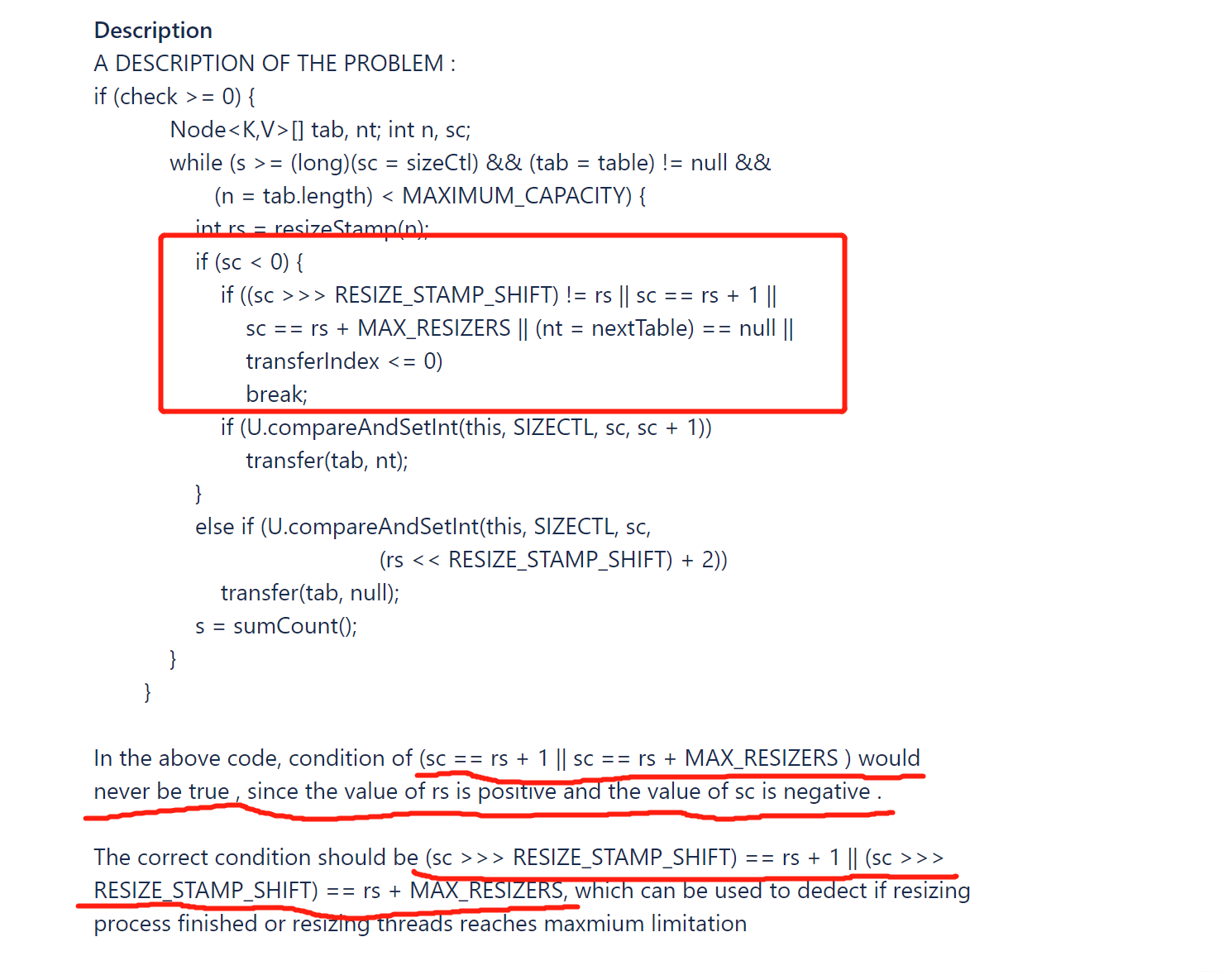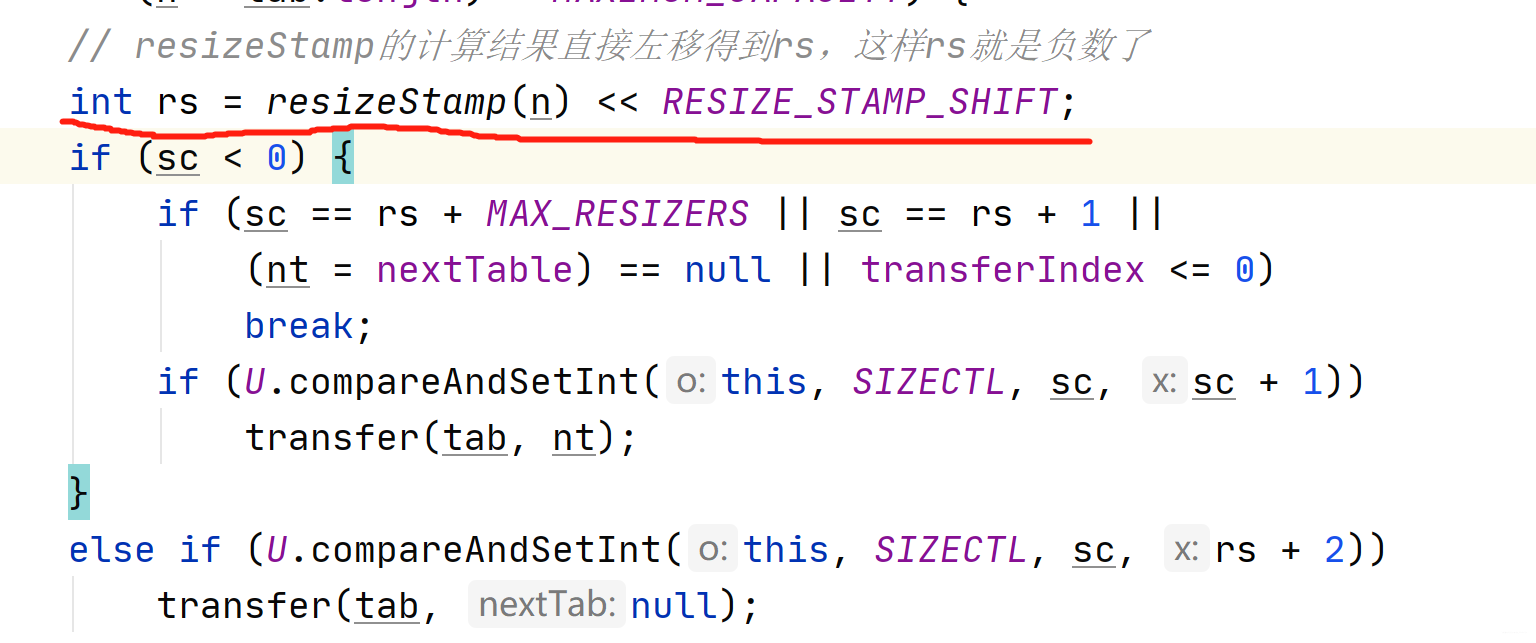#### （3）transfer 元素迁移

private final void transfer(Node<K,V>[] tab, Node<K,V>[] nextTab) {    int n = tab.length, stride;    // 单核不拆分，下面讨论多核的情况    // 计算步长，拆分任务n >>> 3 = n / 2^3    // 先将n分为8份，然后等分给每个cpu，若最后计算的步长小于最小步长16，则设置为16    if ((stride = (NCPU > 1) ? (n >>> 3) / NCPU : n) < MIN_TRANSFER_STRIDE)        stride = MIN_TRANSFER_STRIDE; // subdivide range    if (nextTab == null) {            // initiating        try {            // 扩容 2倍            @SuppressWarnings("unchecked")            Node<K,V>[] nt = (Node<K,V>[])new Node<?,?>[n << 1];            nextTab = nt;        } catch (Throwable ex) {      // try to cope with OOME            sizeCtl = Integer.MAX_VALUE;            return;        }        nextTable = nextTab;        // transferIndex 记录迁移进度        transferIndex = n;    }    int nextn = nextTab.length;    ForwardingNode<K,V> fwd = new ForwardingNode<K,V>(nextTab);    // 从后面的迁移逻辑看到 迁移复制元素是逆序迁移    // advance= true 则代表可继续向前一个位置迁移复制元素    boolean advance = true;    // 是否所有线程都全部迁移完毕，true则可以将nextTab赋值给table了    boolean finishing = false; // to ensure sweep before committing nextTab    // i 代表当前线程正在迁移的数组位置，bound代表它本次可以迁移的范围下限    for (int i = 0, bound = 0;;) {        Node<K,V> f; int fh;        while (advance) {            int nextIndex, nextBound;           // （1）两种情况不需要继续向前一个位置迁移复制元素（逆序）：            // ①i每次自减1，i>=bound说明本批次迁移未完成，不需要继续向前推进。            // ②finishing标志为true，说明所有线程分配的迁移任务都已经完成了，则不需要向前推进。            // 若 --i < bound，说明当前批次的迁移任务完成，可继续分配新范围的任务            // 也就是一个线程可以多次分到任务，能者多劳。            if (--i >= bound || finishing)                // 向前一个位置迁移复制元素                advance = false;             //(2) 每次执行，都会把 transferIndex 最新的值同步给 nextIndex            else if ((nextIndex = transferIndex) <= 0) {                //若 transferIndex小于等于0，则说明原数组中所有位置的迁移任务都分配完毕（不代表所有位置都迁移完毕）                //于是，需要跳出while循环，并把 i设为 -1，                // 以跳到（4）判断正在处理的线程是否完成自己负责范围内迁移工作。                i = -1;                advance = false;            }            else if (U.compareAndSwapInt                     (this, TRANSFERINDEX, nextIndex,                      nextBound = (nextIndex > stride ?                                   nextIndex - stride : 0))) {                //（3）cas 设置TRANSFERINDEX，分配任务范围[nextBound,nextIndex)，任务的长度是stride                // 举例，假设 n=64，即初始的transferIndex=64，stride=16                // nextIndex=transferIndex=64，nextBound=nextIndex-stride=48                // bound=48                // i=63                // 从后往前复制                bound = nextBound;                i = nextIndex - 1;                advance = false;  // 本次任务分配完成，结束循环            }        }        // （4）i已经越界了，整个数组的迁移任务已经全部分配完毕        if (i < 0 || i >= n || i + n >= nextn) {            int sc;            if (finishing) {                // 扩容完毕                // nextTable置为空                nextTable = null;                // 新数组赋值给旧数组                table = nextTab;                // sizeCtl 设置为新的数组长度的 3/4.即 3/4 *2n                sizeCtl = (n << 1) - (n >>> 1);                return;            }            // 到这，说明所有的迁移任务都分配完了            // 当前线程也已经完成了自己的迁移任务（无论参与了几次迁移），            // 则sc-1，表明参与扩容的线程数减1            if (U.compareAndSwapInt(this, SIZECTL, sc = sizeCtl, sc - 1)) {                // 迁移开始时，会设置 sc=(rs << RESIZE_STAMP_SHIFT) + 2                // 每当有一个线程参与迁移，sc 就会加 1。                // 因此，这里就是去校验当前 sc 是否和初始值相等。                if ((sc - 2) != resizeStamp(n) << RESIZE_STAMP_SHIFT)                    // 不相等，当前线程扩容任务结束。                    return;                // 相等，说明还有一个线程还在扩容迁移（不一定是触发扩容的第一个线程）                // 则当前线程会从后向前检查一遍，哪些位置的节点没有复制完，就帮忙一起复制。                // 一圈扫描下来，肯定是全部迁移完毕了，则finishing可提前设置为true。                finishing = advance = true;                i = n; // recheck before commit            }        }        else if ((f = tabAt(tab, i)) == null)            // （5）若i的位置元素为空，就把占位节点设置为fwd标志。            // 设置成功，advance置为true，向前推进复制             advance = casTabAt(tab, i, null, fwd);        else if ((fh = f.hash) == MOVED)            // （6）若当前位置的头结点是 ForwardingNode ，则说明这个位置的所有节点已经迁移完成，            // 可以继续向前迁移复制其他位置的节点            advance = true; // already processed        else {            // （7）对tab[i]进行迁移，可能是链表 or 红黑树            synchronized (f) {                if (tabAt(tab, i) == f) {                    Node<K,V> ln, hn;                    if (fh >= 0) {                        // 链表                        int runBit = fh & n;                        Node<K,V> lastRun = f;                        // lastRun并不是一条链表的最后一个，一条链表的节点可以分为两类，                        // 在循环中寻找lastRun的满足条件是链表中最后一个与前一个节点runBit不相等的节点作为lastRun，                        // 而此时lastRun后面可能还有节点，但runBit都是和lastRun相等的节点。                        // 这里找lastRun和java7是一样的                        for (Node<K,V> p = f.next; p != null; p = p.next) {                            // 计算p的位置                            int b = p.hash & n;                            if (b != runBit) {                                // 和runBit不是同一位置                                runBit = b;                                lastRun = p;                            }                        }                        // hash & n=0为低位节点，hash & n!=0为高位节点。                        // 判断找到的lastRun是低位节点还是高位节点                        if (runBit == 0) {                            ln = lastRun;                            hn = null;                        }                        else {                            hn = lastRun;                            ln = null;                        }                        // lastRun之前的结点因为fh&n不确定，所以全部需要再hash分配。                        for (Node<K,V> p = f; p != lastRun; p = p.next) {                            int ph = p.hash; K pk = p.key; V pv = p.val;                            if ((ph & n) == 0)                                ln = new Node<K,V>(ph, pk, pv, ln);                            else                                hn = new Node<K,V>(ph, pk, pv, hn);                        }                        setTabAt(nextTab, i, ln);                        setTabAt(nextTab, i + n, hn);                        setTabAt(tab, i, fwd);                        advance = true;                    }                    else if (f instanceof TreeBin) {                        // 是红黑树，                        // 原理上和链表迁移的过程差不多，也是将节点分成高位节点和低位节点                        TreeBin<K,V> t = (TreeBin<K,V>)f;                        // lo低位树头节点，loTail低位树尾节点                        // hi高位树头节点，hiTail高位树尾节点                        TreeNode<K,V> lo = null, loTail = null;                        TreeNode<K,V> hi = null, hiTail = null;                        int lc = 0, hc = 0;                        for (Node<K,V> e = t.first; e != null; e = e.next) {                            int h = e.hash;                            TreeNode<K,V> p = new TreeNode<K,V>                                (h, e.key, e.val, null, null);                            if ((h & n) == 0) {                                if ((p.prev = loTail) == null)                                    lo = p;                                else                                    loTail.next = p;                                // 尾插法                                loTail = p;                                ++lc;                            }                            else {                                if ((p.prev = hiTail) == null)                                    hi = p;                                else                                    hiTail.next = p;                                hiTail = p;                                ++hc;                            }                        }                        // 低位节点的个数 <= UNTREEIFY_THRESHOLD=6, 则树退为链表                        // 否则判断是否有高位节点，无，则原先那棵树t就是一棵低位树，直接赋值给ln                        // 有高位节点，则低位节点重新树化。                        // 高位节点的判断同理                        ln = (lc <= UNTREEIFY_THRESHOLD) ? untreeify(lo) :                            (hc != 0) ? new TreeBin<K,V>(lo) : t;                        hn = (hc <= UNTREEIFY_THRESHOLD) ? untreeify(hi) :                            (lc != 0) ? new TreeBin<K,V>(hi) : t;                        setTabAt(nextTab, i, ln);                        setTabAt(nextTab, i + n, hn);                        setTabAt(tab, i, fwd);                        advance = true;                    }                }            }        }    }}

##### （3.1）多线程间是如何分配任务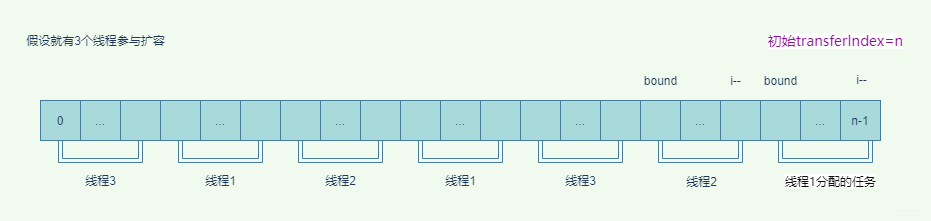transferIndex记录迁移的进度，初始为原数组的长度 n，逆序进行。ForwardingNode转发节点，某个位置的元素迁移完了但是整个迁移任务还没结束，这个位置就会被ForwardingNode占位，写线程遇到ForwardingNode会帮助扩容，读线程遇到ForwardingNode转发请求。

transferIndex<=0时，表示所有任务分配完毕，且分给当前线程的迁移任务都做完了，则 i 置为-1。此时当前线程也没必要再帮助扩容了，则扩容线程数-1（sc - 1）。但是这里有个点，作者想的很缜密，当还有一个线程的迁移任务没有做完时（(sc - 2) = resizeStamp(n) << RESIZE_STAMP_SHIFT），此时倒数第二个线程自己的任务做完了，并不会立即停止，而是从后向前再遍历检查一遍，是否所有位置的节点都迁移完了，还没有开始迁移的位置则迁移，所以倒数第二个线程可能会帮助最后一个线程做任务，一圈扫描下来，可确保所有位置的元素都迁移完了，此时就可以将新数组nextTab替换旧数组table，同时设置新的扩容阈值给sizeCtl

##### （3.2）将元素从旧数组迁移到新数组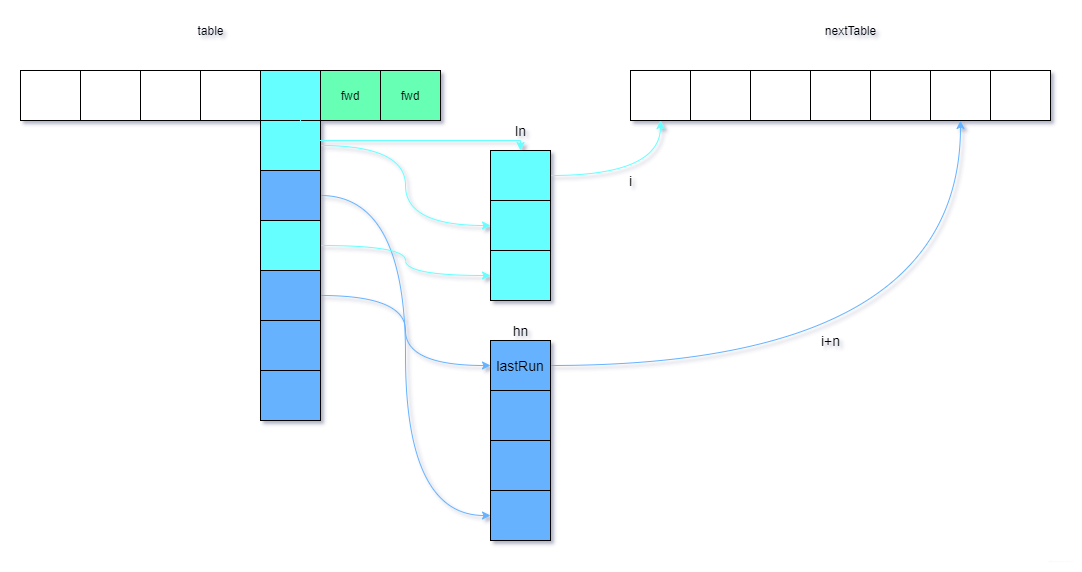java8 依然用了lastRun的概念，同时也用了高低位节点的概念，将一条链表或红黑树分为两种节点，即两条链表，高位节点链表和低位节点链表。那是如何区分的呢？

hash & n=0为低位节点，hash & n!=0为高位节点。低位节点迁移到新数组时位置不会变，原来在旧数组的位置为 i，到新数组还是 i；而高位节点，原来在数组的位置为 i，到新数组的位置则为 i+旧数组的长度 n。为什么有这个规律呢？

n 的掩码 mask=n-1，16 的掩码 1111，32 的掩码 11111，2n 的掩码比 n 的掩码多一位 1，十进制上多 n。mask&ph 起到模运算的效果来映射数组下标：

• ph & n=0时， ph的第 m 位为 0，是无效位，所以ph&(n-1)一定等于ph&(2n-1)

• ph & n=n时， ph的第 m 位是 1，是有效位，ph&(2n-1)的第 m 位为 1，其余位和ph&(n-1)一样，所以ph&(n-1)+n=ph&(2n-1)

### 2、helpTransfer 帮助扩容

final Node<K,V>[] helpTransfer(Node<K,V>[] tab, Node<K,V> f) {    Node<K,V>[] nextTab; int sc;    // 判断当前节点f是否为ForwardingNode，且其转发的nextTable是否为空    if (tab != null && (f instanceof ForwardingNode) &&        (nextTab = ((ForwardingNode<K,V>)f).nextTable) != null) {        int rs = resizeStamp(tab.length) << RESIZE_STAMP_SHIFT;        while (nextTab == nextTable && table == tab &&               (sc = sizeCtl) < 0) {            // 新数组nextTable，旧数组table地址均未改变，且sc<0，            // 则判断扩容线程数是否达到最大值sc == rs + MAX_RESIZERS            // 扩容是否结束sc == rs + 1还没来得及commit（table=nextTable）            // 任务是否分配完毕 transferIndex <=0            if (sc == rs + MAX_RESIZERS || sc == rs + 1 ||                transferIndex <= 0)                break;            // 需要帮助扩容，扩容线程数+1            if (U.compareAndSetInt(this, SIZECTL, sc, sc + 1)) {                transfer(tab, nextTab);                break;            }        }        return nextTab;    }    return table;}

### 3、tryPresize 链表转红黑树触发扩容

tryPresize有可能是putAll调用的，参数 size 就是添加的 map 的 size，当数组 table 还没初始化时触发初始化，根据 size 和扩容因子计算的预估容量 c（tableSizeFor详解以及初始容量优化见上一篇文章）和原 table 的初始容量 sc 比较，谁大谁则成为新初始容量。

tryPresizetreeifyBin调用，tryPresize(n << 1)，为确保因达到转为红黑树阈值但数组长度小于 64 情况下一定要触发扩容，所以传给tryPresize的参数是数组长度的 2 倍，使得计算的 c 一定大于扩容阈值，但是只传个 n 也是一定大于扩容阈值的，不太能 get 到作者为什么要传个n<<1

private final void tryPresize(int size) {    // c 计算合适的扩容 容量，size >= MAXIMUM_CAPACITY/2 则合适的容量是MAXIMUM_CAPACITY    // 否则找一个>=size且离size最近的2的整数次方的值    int c = (size >= (MAXIMUM_CAPACITY >>> 1)) ? MAXIMUM_CAPACITY :        tableSizeFor(size + (size >>> 1) + 1);    int sc;    while ((sc = sizeCtl) >= 0) {        Node<K,V>[] tab = table; int n;        if (tab == null || (n = tab.length) == 0) {            // tryPresize 在 putAll里调用时，如果数组还未初始化，则进行数组初始化            // putAll 时 size传的是 需要添加的map的size，c是根据size计算的一个预估容量值，为的是避免不必要的扩容            // 预估值c和原数组初始容量谁大，谁就是新的初始容量            n = (sc > c) ? sc : c;            if (U.compareAndSetInt(this, SIZECTL, sc, -1)) {                try {                    if (table == tab) {                        @SuppressWarnings("unchecked")                        Node<K,V>[] nt = (Node<K,V>[])new Node<?,?>[n];                        table = nt;                        sc = n - (n >>> 2);                    }                } finally {                    sizeCtl = sc;                }            }        }        // table不为空且有数据        // putAll 计算的c可能大于sc扩容阈值，则会先触发扩容，则批量添加元素。        // 而treeifyBin 传进来的参数为n<<1，是一定大于扩容阈值sc的，所以是一定会触发扩容的        else if (c <= sc || n >= MAXIMUM_CAPACITY)            break;        else if (tab == table) {            // 这里java12和java8不太一样了            // java8 还会判断 是第一个触发扩容还是帮助扩容            // 而java12认为是第一个线程触发扩容，若cas失败，则说明有其他线程触发，也不帮助。            int rs = resizeStamp(n);            if (U.compareAndSetInt(this, SIZECTL, sc,                                    (rs << RESIZE_STAMP_SHIFT) + 2))                transfer(tab, null);        }    }}

## 五、get 操作遇到正在扩容

java8 扩容引入 fwd 也是和 java7 扩容的一个大区别，java7 是一个segment内的HashEntry数组完全迁移完，再将新数组替换旧数组，只能保证最终一致性，get 操作是不会感知数组正在扩容的，其他操作也是感知不到的，所以也就不会有帮助扩容的概念。

public V get(Object key) {    Node<K,V>[] tab; Node<K,V> e, p; int n, eh; K ek;    // 1. hash高低位扰动    int h = spread(key.hashCode());    // 2.hash映射找到对应数组中的槽    if ((tab = table) != null && (n = tab.length) > 0 &&        (e = tabAt(tab, (n - 1) & h)) != null) {        if ((eh = e.hash) == h) {            // 3.如果 槽中的占位节点就是要找的key，则返回            if ((ek = e.key) == key || (ek != null && key.equals(ek)))                return e.val;        }        // 4.eh < 0 可能遇到 红黑树 or ForwardingNode        else if (eh < 0)            return (p = e.find(h, key)) != null ? p.val : null;        // 5. 否则就是普通的链表 遍历寻找        while ((e = e.next) != null) {            if (e.hash == h &&                ((ek = e.key) == key || (ek != null && key.equals(ek))))                return e.val;        }    }    return null;}

static final class ForwardingNode<K,V> extends Node<K,V> {    final Node<K,V>[] nextTable;    ForwardingNode(Node<K,V>[] tab) {        super(MOVED, null, null, null);        this.nextTable = tab;    }    // 转发到nextTable中继续检索    Node<K,V> find(int h, Object k) {        // loop to avoid arbitrarily deep recursion on forwarding nodes        outer: for (Node<K,V>[] tab = nextTable;;) {            Node<K,V> e; int n;            if (k == null || tab == null || (n = tab.length) == 0 ||                (e = tabAt(tab, (n - 1) & h)) == null)                // 新数组 映射的槽是空的则返回null                return null;            for (;;) {                int eh; K ek;                if ((eh = e.hash) == h &&                    ((ek = e.key) == k || (ek != null && k.equals(ek))))                    return e;                if (eh < 0) {                    if (e instanceof ForwardingNode) {                        tab = ((ForwardingNode<K,V>)e).nextTable;                        // 又遇到另一个转发节点，跳过一次外围循环，从新的tab检索，                        // 不会在扩容阶段又在新数组上扩容把？有待后续验证                        continue outer;                    }                    else                        // 这里就是红黑树了，去树上找                        return e.find(h, k);                }                if ((e = e.next) == null)                    // 到最后了还没找到则返回null                    return null;            }        }    }}

## 六、replaceNode 更新节点

removereplace都是调用replaceNode方法。remove调用时，传入的valuecv都是 null，若遍历节点找到 key 相等的节点则会将该节点删除。

/** * Implementation for the four public remove/replace methods: * Replaces node value with v, conditional upon match of cv if * non-null.  If resulting value is null, delete. * @param key 需要替换的key * @param value 替换的新值 * @param cv 旧值 * @return */final V replaceNode(Object key, V value, Object cv) {    int hash = spread(key.hashCode());    for (Node<K,V>[] tab = table;;) {        Node<K,V> f; int n, i, fh;        if (tab == null || (n = tab.length) == 0 ||            (f = tabAt(tab, i = (n - 1) & hash)) == null)            // 1.哈希映射位置的节点为null，则不需要替换            break;        else if ((fh = f.hash) == MOVED)            // 2.fh=MOVED，正在扩容，则帮助扩容            tab = helpTransfer(tab, f);        else {            V oldVal = null;            boolean validated = false;            synchronized (f) {                if (tabAt(tab, i) == f) {                    if (fh >= 0) {                        // 普通链表替换                        validated = true;                        for (Node<K,V> e = f, pred = null;;) {                            K ek;                            if (e.hash == hash &&                                ((ek = e.key) == key ||                                 (ek != null && key.equals(ek)))) {                                // key和hash相等                                // cv 不为null，需要判断cv和ev是否相等，相等才能替换 or 删除                                V ev = e.val;                                if (cv == null || cv == ev ||                                    (ev != null && cv.equals(ev))) {                                    oldVal = ev;                                    if (value != null)                                        // 传入的value!=null，则替换旧值为新值                                        e.val = value;                                    else if (pred != null)                                        // 传入的value=null，且pred前驱不为null，则删除该节点                                        pred.next = e.next;                                    else                                        // 前驱为null，说明删除的是头节点                                        setTabAt(tab, i, e.next);                                }                                // 已经找到节点并对其做了处理，结束循环                                break;                            }                            // 继续遍历                            pred = e;                            if ((e = e.next) == null)                                // 下一个节点为null,说明到尾部了，结束循环，需要替换的节点不存在                                break;                        }                    }                    else if (f instanceof TreeBin) {                        // 红黑树 替换                        validated = true;                        TreeBin<K,V> t = (TreeBin<K,V>)f;                        TreeNode<K,V> r, p;                        if ((r = t.root) != null &&                            (p = r.findTreeNode(hash, key, null)) != null) {                            // r.findTreeNode 从红黑树中找到节点                            V pv = p.val;                            if (cv == null || cv == pv ||                                (pv != null && cv.equals(pv))) {                                // 传入cv旧值，则需要判断                                oldVal = pv;                                if (value != null)                                    // 传入的value不为null,则替换                                    p.val = value;                                // 传入的value为null,则从树中删除节点                                else if (t.removeTreeNode(p))                                    // 删除节点时，不会判断树上的节点的数量是否被减到退化为链表的阈值UNTREEIFY_THRESHOLD                                    // t.removeTreeNode(p) 返回结果并不是删除是否成功，                                    // 而是true表示树很小了（根节点为null or 左孩子or右孩子为null），需要退化成链表                                    setTabAt(tab, i, untreeify(t.first));                            }                        }                    }                }            }            if (validated) {                // validated=true，前面普通链表or红黑树中已经对该节点做了处理                if (oldVal != null) {                    if (value == null)                        // value=null是删除节点，count-1                        addCount(-1L, -1);                    return oldVal;                }                break;            }        }    }    return null;}

## 七、总结

1、第一个触发扩容的线程计数时为什么是+2？+2 的话，就可以用sc==rs+1来判断所有线程都扩容完了，但是第一个线程+1，也可以用sc==rs来判断所有的线程都扩容完了。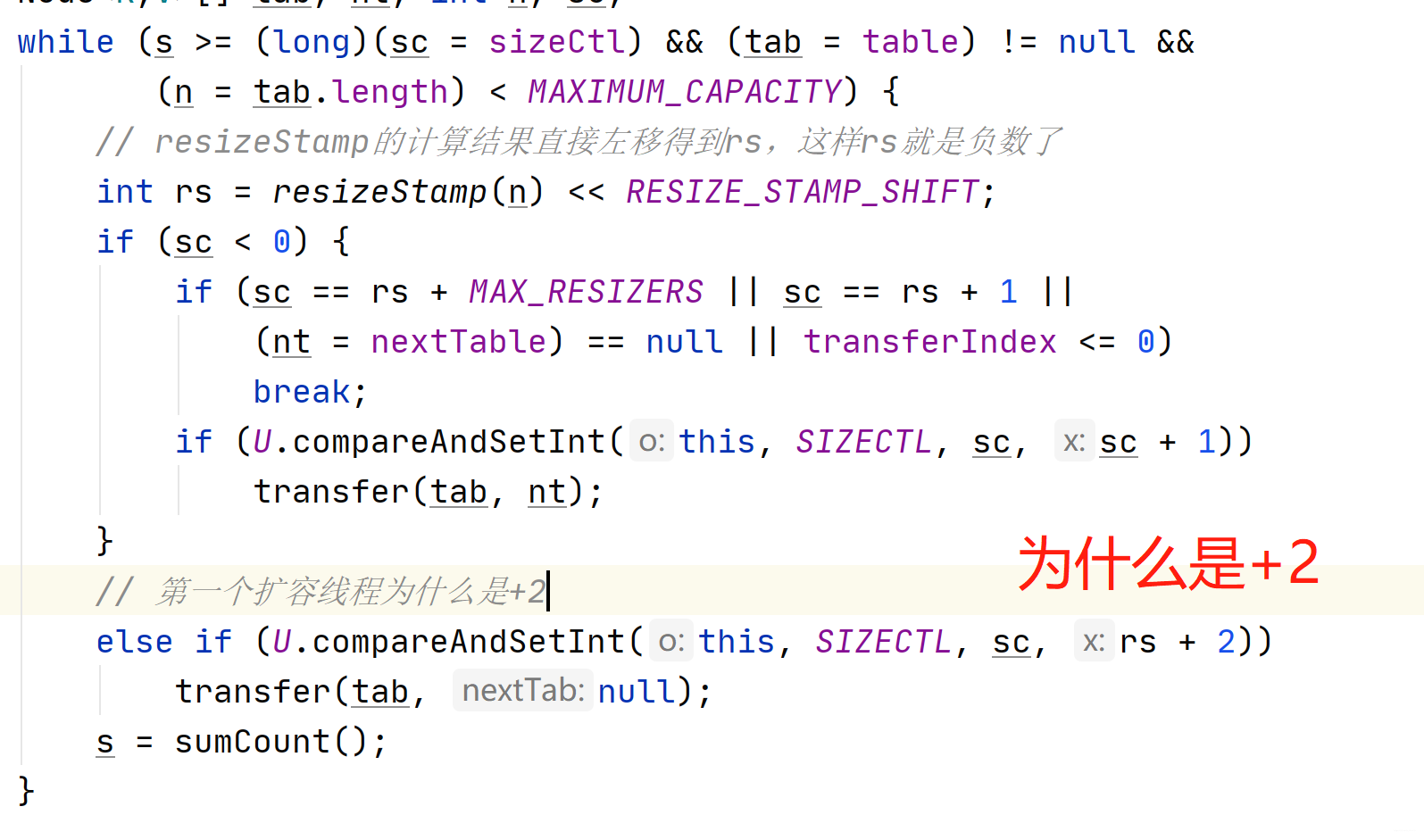2、treeifyBin想树化却数组长度没达到 64，就调用tryPresize扩容，但是为什么参数是 n<<1，数组长度的 2 倍？如果是为了确保调用tryPresize一定能触发扩容，传个 n 也是一定大于当前扩容阈值的呀。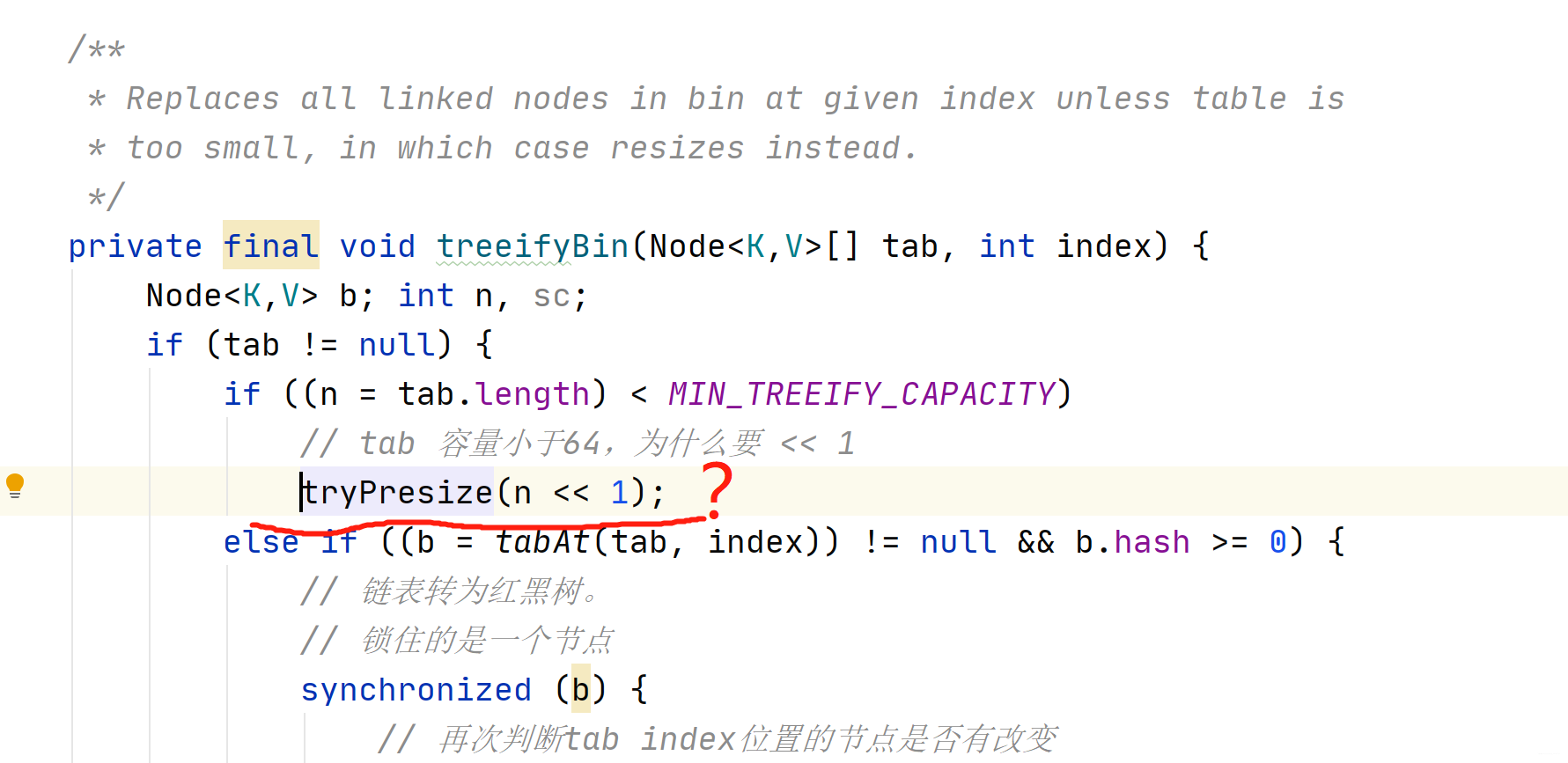(ConcurrentHashMap的源码远不止于此，但是有些函数不常用，甚至都没用过，就暂时先不去研究了。)

PS: 如若文章中有错误理解，欢迎批评指正，同时非常期待你的评论、点赞和收藏。我是徐同学，愿与你共同进步！## 评论